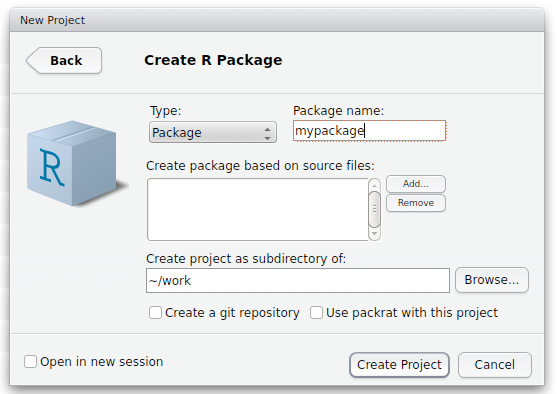## 21 Feb 2018, Research Bazaar, University of Melbourne

Damjan Vukcevic
Lecturer in Statistical Genomics

## Why R packages?

• Easier to manage

• Easier to reuse

• Easier to share

## Overview

1. What is an R package?

3. Developing R packages using RStudio

## What is an R package?

"Packages are the fundamental units of reproducible R code." (Hadley Wickham)

"R packages are the best way to distribute R code and documentation…" (Karl Broman)

## R functions (refresher)

Functions are an even more 'fundamental unit' than packages

The simplest way to 'package up' some R code

## Example

Define a function:

``````# Calculate the square of x.
square <- function(x)
x^2
``````

Use the function:

``````square(1:3)
``````
``````##  1 4 9
``````

## An R package is…

…a standard way to 'package up' R functions together

R code ➔ functions ➔ packages

## `functions.R`, the poor man's R package

1. Place function definitions in a file called `functions.R`

2. Load this in other scripts using `source("functions.R")`

## Examples

In `functions.R`:

``````# Calculate the square of x.
square <- function(x)
x^2
``````

``````source("functions.R")
square(1:3)
``````
``````##  1 4 9
``````

## Examples

In `functions.R`:

``````# Resample values from a given vector.
resample <- function(x, ...)
x[sample.int(length(x), ...)]
``````

``````source("functions.R")
resample(letters[1:10])
``````
``````##   "c" "h" "d" "a" "i" "e" "g" "j" "f" "b"
``````

## Note: only need to `source()` once

``````source("functions.R")
square(1:3)
``````
``````##  1 4 9
``````
``````resample(letters[1:10])
``````
``````##   "d" "i" "c" "e" "f" "g" "h" "a" "j" "b"
``````

Hilary Parker:

I really should just make an R package with these functions so I don't have to keep copy/pasting them like a goddamn luddite.

Create folder structure

``````mypackage/
└── R/
``````

Move `functions.R` into the `R` subfolder

``````mypackage/
└── R/
└── functions.R
``````

Create the `DESCRIPTION` and `NAMESPACE` files

``````mypackage/
├── R/
│   └── functions.R
├── DESCRIPTION
└── NAMESPACE
``````

Note: RStudio will automatically create these files

Your `DESCRIPTION` file should look similar to:

``````Package: mypackage
Title: This is my first R package
Description: This package was created as a way to learn how to write
R packages.
Authors@R: person("Damjan", "Vukcevic", email = "damjan@vukcevic.net",
role = c("aut", "cre"))
Version: 0.1
Depends: R (>= 3.3.1)
LazyData: true
``````

Your `NAMESPACE` file should look like:

``````# Export all names
exportPattern("^[[:alpha:]]+")
``````

Now, let's do all of this in RStudio…

## RStudio: Starting a new package

1. File > New Project… > New Directory > R Package > (dialog box) > Create Project2. Edit the `DESCRIPTION` file

## RStudio: Working on your package

2. Build > Build and Reload
(Ctrl/Cmd + Shift + B)
3. Try running your functions in the Console
4. Repeat until happy

Simply use `library(mypackage)` instead of `source("functions.R")`

For example:

``````library(mypackage)
square(1:3)
``````
``````##  1 4 9
``````
``````resample(letters[1:10])
``````
``````##   "f" "b" "a" "c" "e" "g" "i" "h" "d" "j"
``````

## Tip for larger packages

You can split up your code into any number of `.R` files

``````mypackage/
├── R/
│   ├── resample.R
│   └── square.R
├── DESCRIPTION
└── NAMESPACE
``````

All `.R` files within the `R` subfolder will automatically be included in your package

(More convenient than if `source()`-ing)

## Benefits of packages (vs `functions.R`)

• Easier to reuse
• Easier to share
• Easier to maintain as complexity increases
• Cleaner project code

And also (not covered today):

• Better documentation
• Easier automated testing
• Dependency and namespace management

## Challenge problems

### Challenge 1

Write an R package called `challenge1` that contains the functions `square()` and `cube()` which respectively square or cube an input number.

### Challenge 2

Write an R package called `challenge2` that contains the functions `fullname()` and `sentence()` that behave as follows:

• `fullname()` combines two input strings into a single string, for example:
`fullname("Damjan", "Vukcevic")` should return `"Damjan Vukcevic"`.

• `sentence()` takes two input strings and returns a sentence, in this form:
`sentence("Damjan", "Vukcevic")` should return ```"The name Damjan Vukcevic contains 14 characters"```.

Hint: use `paste()` and `nchar()`